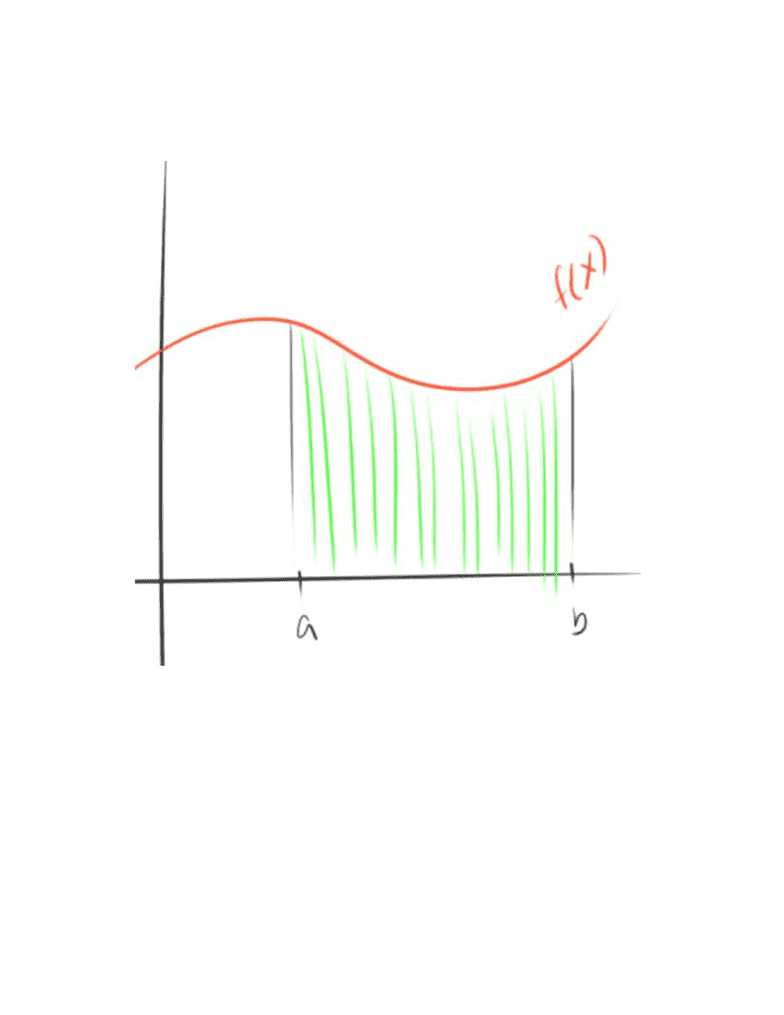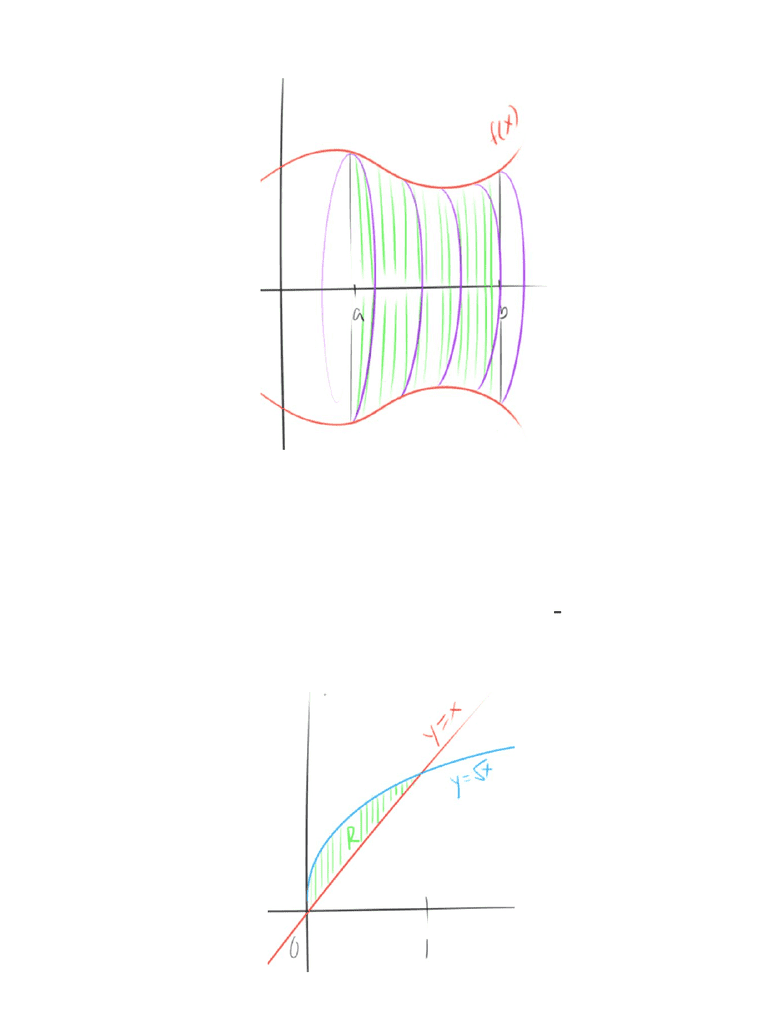Study Guides (390,000)
US (220,000)
UC-Irvine (3,000)
MATH (600)
MATH 2B (100)
All (60)
Midterm

# MATH 2B Midterm: MATH2B, Chapter 6.2 term test 1Premium

Department
Mathematics
Course Code
MATH 2B
Professor
All
Study Guide
Midterm

This preview shows page 1. to view the full 5 pages of the document.It’s the sum of the area of infinite rectangles under the function
What is the area of each rectangle?
(xxf
Therefore
rea (x)dxA =
b
a
f
What then, is the volume of the area under the curve rotated around a line?
What is the Area Under the Curve?

Unlock to view full version

Subscribers Only

Only page 1 are available for preview. Some parts have been intentionally blurred.

Subscribers Onlyπ (R(x) (x)) dxV =
b
a
2r2
Because the area of a circle is , we can compile an integral from this by usingrπ2
(x) r(x)R2 2
Example #1
Find the volume of a solid with a base of region R between , which and y xy = x=
has cross-sections perpendicular to the x-axis in the shape of squares